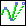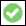> > Change a 3D spline curve

Change a 3D spline curve
In a 3D spline curve, you can change the position of the interpolation or control points, insert points, remove points, or extend the spline curve at either end. Open spline curves can be closed and closed spline curves can be opened. You can change the start and end positions or the shape of the curve with respect to existing geometry using the Curve connect condition.
For finer control over the shape of the spline curve, you can attach or modify a tangent vector at any interpolation point. If a tangent vector already exists at a point, you can remove it by clicking the ChangeTan option for the selected point. You can also use the Curve connect condition to specify the tangent of the curve at the start or end point.
To open a closed curve:
Select Position in EndCondition.
Select the section to open. The section is deleted, the new end points become fixed points, and the rest of the curve remains unchanged.
To close an open curve:
Select Closed in EndCondition.
To change a 3D spline curve
1. Click 3D Geometry and then, in the 3D Curve group, clickModify Spline. The Modify Spline dialog box opens.
2. Click the spline curve to modify.
3. Choose a Mode: Interpolation or Control. Control mode will delete information about fix points and tangents.
4. Use move, delete, append, and insert to manipulate the interpolation/control points of the spline curve. The Point 3D CoPilot is used in all position options.
Move: Move a control or interpolation point on the spline curve. If Follow Me is on, the Point 3D CoPilot handle is positioned at the selected interpolation point.
Delete: Delete a control or interpolation point from the spline curve.
Append: Add a new control or interpolation point to the spline curve at the end the curve was originally terminated. If Follow Me is on, the Point 3D CoPilot handle is positioned at the selected end of the curve and repositioned every time a new position is entered. When you append to a Tangential or curvature continuous condition, the interpolation point has a local influence and changes to a fixed point.
Insert: Insert a new control or interpolation point between consecutive points on the spline curve. If Follow Me is on, the Point 3D CoPilot handle is positioned at an interpolation point of the selected segment.
Change Tan: Modify the direction of a tangent vector at an interpolation point on the spline curve. (Does not apply to spline control points.) The Vector 3D CoPilot displays the weight of the tangent. Move the tool to change the tangent weight and direction.
Delete Tan: Delete the tangent at an interpolation point.
5. Choose an End Condition:
Position: No special conditions are set.
Closed: Creates a closed periodic curve. You cannot append a closed curve.
Edge: Define the position and start or end condition of the curve in relation to an existing edge.
Face: Define the position and start or end condition of the curve in relation to an existing face.
Use the Curve connect condition to specify various conditions for the curve.
6. Click Fix Points to limit the influence of the modification.
7. Click Add Int Pt to add one or more interpolation points.
8. Clickto complete the operation.